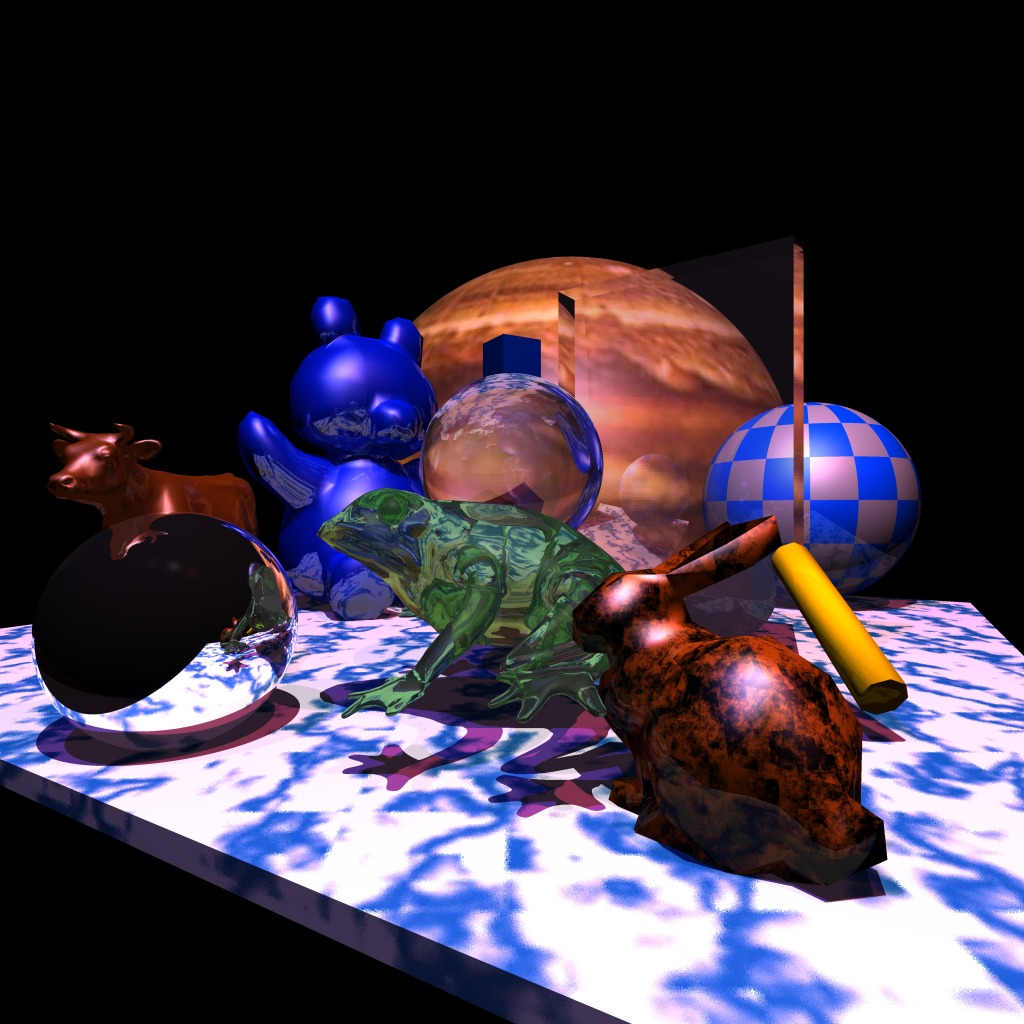# CS 636 Extra Credit

## Summary

For extra credit I added 2d image textures(Jupiter texture on large sphere) and 3d improved Perlin noise based textures of two kinds, turbulence used for a marble texture(on floor) and a fractional Brownian motion texture used on the bunny model. I also added a procedural 2d checkered texture seen on the sphere on the right of the scene.

This scene shows refractions, reflections, and shadows with up to a recursive depth of 8, in addition to the primary ray.

The specular calculations use the Hall lighting model.

Rendering uses BVH acceleration and adaptive supersampling with two levels of subdivision.

Rendering time using for 1024x1024 image on my M1 Mac Mini was 20,820 seconds, far slower than previous assignnments.

## Scene Setup

The camera for the scene is setup at (0,0,4) for the eye point, with a view direction of (0,0,-1) and a view up direction of (0, 1,0 ). The view plane distance is 6, and the horizontal camera angle is PI / 6.

The scene has three point light sources:

• a white light at (0, 15, 5)
• a redish light color=(0.5, 0.1, 0.2) at ( 5, 15, 5)
• a blueish light color=(0.2, 0.1, 0.5) at ( -5, 15, 5)

The scene has a floor (made from a scaled cube) underneath to provide a surface for shadows. The other objects are five spheres, a cube, a scaled transmissive cube, a green transmissive frog, a mesh cylinder, a bunny, a cow, and a teddy bear./BVH

```                Bunny ID=1
transform[
translate=[1.4, -1, 0]
scale=[2, 2, 2]
rotate=[0, -1.0472, 0]
]
surface[
colors diffuse=(0.8, 0.2, 0.9),  ambient=(0.8, 0.2, 0.9),  specular=(1, 1, 1)
coeff. diffuse=0.5,  ambient=0.1,  specular=0.7
specular factor=30
reflection=0.1
]

Sphere 1 ID=2
center=[3, -1.1, -5]
surface[
colors diffuse=(0.0117647, 0.32549, 0.592157),  ambient=(1, 1, 1),  specular=(1, 1, 1)
coeff. diffuse=0.8,  ambient=0.1,  specular=0.4
specular factor=25
reflection=0.1
]

Sphere 2 ID=3
center=[-2.9, -1, -1.5]
surface[
colors diffuse=(1, 1, 1),  ambient=(1, 1, 1),  specular=(1, 1, 1)
coeff. diffuse=0.05,  ambient=0.05,  specular=0.1
specular factor=999
reflection=1
]

Sphere 3 ID=4
center=[2, 1, -20]
surface[
colors diffuse=(0.470588, 0.988235, 0.54902),  ambient=(1, 1, 1),  specular=(1, 1, 1)
coeff. diffuse=0.8,  ambient=0.1,  specular=0.4
specular factor=25
reflection=0.5
]

Sphere 4 ID=5
center=[6, 0.2, -9]
surface[
colors diffuse=(1, 1, 1),  ambient=(1, 1, 1),  specular=(1, 1, 1)
coeff. diffuse=0.8,  ambient=0.1,  specular=0.4
specular factor=25
reflection=0.1
]

Sphere 5 ID=6
center=[0, 0.5, -3]
surface[
colors diffuse=(1, 1, 1),  ambient=(1, 1, 1),  specular=(1, 1, 1)
coeff. diffuse=0.05,  ambient=0.05,  specular=0.1
specular factor=25
reflection=0
]

Teddy ID=7
transform[
translate=[-3, 1, -9]
scale=[0.15, 0.15, 0.15]
rotate=[0, 0.785398, 0]
]
surface[
colors diffuse=(0, 0.117647, 1),  ambient=(0, 0.117647, 1),  specular=(1, 1, 1)
coeff. diffuse=0.5,  ambient=0.1,  specular=0.5
specular factor=25
reflection=0.3
]

Cow ID=8
transform[
translate=[-3.3, -0.3, -2.2]
scale=[3.2, 3.2, 3.2]
rotate=[0, -1.5708, 0]
]
surface[
colors diffuse=(0.909804, 0.388235, 0.0392157),  ambient=(0.909804, 0.388235, 0.0392157),  specular=(1, 1, 1)
coeff. diffuse=0.3,  ambient=0.1,  specular=0.6
specular factor=15
reflection=0.1
]

Cylinder ID=9
transform[
translate=[3, -1, -2]
scale=[1, 1, 1]
rotate=[0.523599, 0, 0]
]
surface[
colors diffuse=(0.988235, 0.85098, 0),  ambient=(0.988235, 0.85098, 0),  specular=(0.988235, 0.85098, 0)
coeff. diffuse=0.8,  ambient=0.05,  specular=0.05
specular factor=2
reflection=0.1
]

Cube ID=10
transform[
translate=[0, 3.5, -11]
scale=[1, 1, 1]
rotate=[0, -1.0472, 0]
]
surface[
colors diffuse=(0, 0.117647, 0.423529),  ambient=(0, 0.117647, 0.423529),  specular=(0, 0.117647, 0.423529)
coeff. diffuse=0.8,  ambient=0.1,  specular=0.4
specular factor=25
reflection=0.1
]

Window ID=11
transform[
translate=[2, 1, -4]
scale=[3, 4, 0.5]
rotate=[0, -0.628319, 0]
]
surface[
colors diffuse=(1, 1, 1),  ambient=(1, 1, 1),  specular=(1, 1, 1)
coeff. diffuse=0.03,  ambient=0.03,  specular=0.03
specular factor=25
reflection=0
]

Frog ID=12
transform[
translate=[-0.1, 0, -2]
scale=[1.3, 1.3, 1.3]
rotate=[0, 0, 0]
]
surface[
colors diffuse=(0.752941, 1, 0.752941),  ambient=(0.752941, 1, 0.752941),  specular=(0.752941, 1, 0.752941)
coeff. diffuse=0.05,  ambient=0.05,  specular=0.1
specular factor=25
reflection=0
]

Floor ID=13
transform[
translate=[0, -2, -6.7]
scale=[14, 0.2, 14]
rotate=[0, -0.523599, 0]
]
surface[
colors diffuse=(1, 1, 1),  ambient=(1, 1, 1),  specular=(1, 1, 1)
coeff. diffuse=0.8,  ambient=0.1,  specular=0.1
specular factor=2
reflection=0
]

```